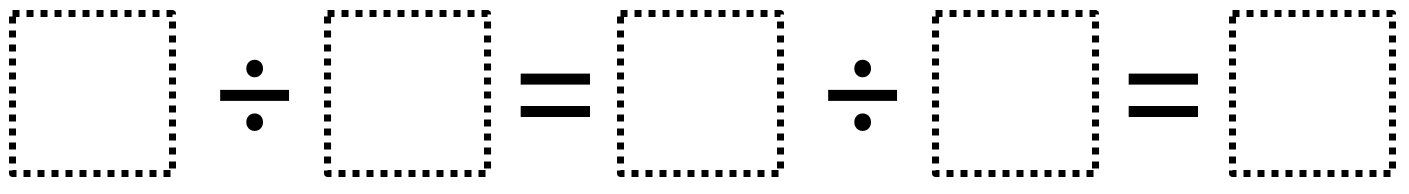# Whole Number Division

Directions: Using the digits 1 to 9 at most one time each, fill in the boxes to make a true statement.### Hint

What possible values can the single digit have?

The only possible answer is 8 ÷ 4 = 6 ÷ 3 = 2

Source: Owen Kaplinsky

## Product Close to 1,000

Directions: Using the digits 1 to 9 at most one time each, place a digit …

1.This really puzzled my students. When we looked at the hint and then the answer, they realized there was a mistake. 8 divided by 4 is 2, not 6.

2.Thanks! What I meant to say was 8/4=2 and 6/3=2.

•That still doesn’t make a true statement, though. Is there something I’m not understanding?

•I think so. Instead of 8/4=2 and 6/3=2, you could say 8/4=6/3=2. Also, you can think of it as three parts. Each one equals the same thing. Part one is 8/4 or 2, part 2 is 6/3, or 2, and part 3 is 2.

3.Some parentheses might help

4.i think it’s 8 4 6 3 2

5.i think it’s 8 4 6 3 2

6.8 divided by 2=4 divided by 2=2

7.i think it might be 8 4 6 3 2

8.i did it wrong its 8 divided by 4 = 2 so the answer is 2

9.Person aka kaleya

8 ÷ 4 = 6 ÷ 3 = 2

10.8 ÷ 4 = 6 ÷ 3 = 2

11.JaliyahSingleton

8 ÷ 4 = 6 ÷ 3 = 2

12.it should be 8/4=2/1=1 because 8/4=6/3=2 and 8/4=2/3=2 cant be true

13.8 ÷ 4 = 6 ÷ 3 = 2 is the answer

14.8 divide 4=6 divide 3=2

15.8/4=6/3=2

16.Perla Martinez

8/4=6/3=6

17.8/4=6/3=2

I made an error the first time with 8/4 = 2/1 = 2 but realized I used 2 twice.

18.lavellas peterson

8/4=6/3=2

19.I am wondering if posting it like this would provide some clarification:
_ / _= _ / _

Then, the answer would be 8/4=6/2.

Couldn’t 8/4=2/1 also be an answer?

•Yeah, that could be another answer.

20.I allowed them to put 2 digits in a line if they wanted to. This helped.

54/9 = 6/3 = 2

21.How is the answer 8/4=2 and 6/3=2 when the question is clearly some number divided by some number equals some number that is divided by some number that equals the answer. You are only able to use numbers 1 to 9. This makes no sense and if anything your are mixing our kids up.